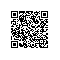# let、const关键字

ES6 之前，JavaScript 中变量默认是全局性的，只存在函数级作用域，声明函数曾经是创造作用域的唯一方法。这点和其他编程语言存在差异，其他语言大多数都存在块级作用域。所以在 ES6 中，新提出的 letconst 关键字使这个缺陷得到了修复。

if (true) {
let a = 'name';
}
console.log(a); // ReferenceError: a is not defined

const MYNAME = 'liangyin';
MYNAME = 'doke';
// TypeError: Assignment to constant variable.
const MYNAME = {
first: 'liang'
};
MYNAME.last = 'yin';
// {first: "liang", last: "yin"}

• 尽量使用 letconst 代替 var
• 声明方法尽量使用 const 以防止后期无意覆盖
• const 定义的变量使用大写形式

# 函数

## 箭头函数

let add = (a, b) => {
return a + b;
}
// 当后面是表达式(expression)的时候，还可以简写成
let add = (a, b) => a + b;
// 等同于
let add = function(a, b) {
return a + b;
}
// 在回调函数中应用
let number = [1, 2, 3];
let doubleNumber = number.map(number => number * 2);
console.log(doubleNumber);
// [2, 4, 6] 看起来很简便吧

## this 在箭头函数中的使用

var age = 2;
var kitty = {
age: 1,
grow: function() {
setTimeout(function() {
console.log(++this.age);
}, 100)
}
};
kitty.grow();
// 3

let kitty = {
age: 1,
grow: function() {
const self = this;
setTimeout(function() {
console.log(++self.age);
}, 100);
}
}
// 或者
let kitty = {
age: 1,
grow: function() {
setTimeout(function() {
console.log(this.age);
}.bind(this), 100)
}
}

let kitty = {
age: 1,
grow: function() {
setTimeout(() => {
console.log(this.age);
}, 100)
}
}

## 函数默认参数

ES6 出现以前，面对默认参数都会让人感到很痛苦，不得不采用各种 hack 手段，比如说：values = values || []。现在一切都变得轻松很多。

function desc(name = 'liangyin', age = 18) {
return name + '已经' + age + '岁了'
}
desc();
// liangyin已经18岁了

## Rest 参数

function test(...args) {
console.log(args);
}
test(1, 2, 3);
// [1,2,3]
function test2(name, ...args) {
console.log(args);
}
test2('liangyin', 2, 3);
// [2,3]

• Rest 参数只是没有指定变量名称的参数数组，而 arguments 是所有参数的集合。
• arguments 对象并不是一个真正的数组，而 Rest 参数是一个真正的数组，可以使用各种数组方法，比如 sortmap等。

# 展开操作符

## 用于函数调用

function test(x, y, z) {};
var args = [1, 2, 3];
test.apply(null, args);

function test(x, y, z) {};
let args = [1, 2, 3];
test(...args);

## 用于数组字面量

var arr1 = [1, 2, 3];
var arr2 = [4, 5, 6];
var arr3 = arr1.concat(arr2);
console.log(arr3);
// [1,2,3,4,5,6]

let arr1 = [1, 2, 3];
let arr2 = [4, 5, 6];
let arr3 = [...arr1, ...arr2];
console.log(arr3);
// [1,2,3,4,5,6]

## 对象的展开运算符（ES7）

let uzi = {
name: 'uzi',
age: 50
};
uzi = {
...uzi,
sex: 'male'
};
console.log(uzi);
// {name: "uzi", age: 50, sex: "male"}

# 模板字符串

ES6 之前的时代，字符串拼接总是一件令人很很很不爽的一件事，但是在ES6的时代，这个痛点终于被治愈了！！！

// 之前的做法
var name = 'uzi';
var a = 'My name is ' + uzi + '!';
// 多行字符串
var longStory = 'This is a long story,' + 'this is a long story,' + 'this is a long story.'
// 有了 ES6 之后我们可以这么做
let name = 'uzi';
let a = My name is ${name} !; let longStory = This is a long story, this is a long story, this is a long story. # 解构赋值 解构语法可以快速从数组或者对象中提取变量，可以用一个表达式读取整个结构。 ## 解构数组 let number = ['one', 'two', 'three']; let [one, two, three] = number; console.log(${one},${two},${three});
// one,two,three

## 解构对象

let uzi = {
name: 'uzi',
age: 20
};
let {
name,
age
} = uzi;
console.log(${name},${age});
// uzi,20

# 类

ES6 中提供了 class 这个语法糖，让开发者模仿其他语言类的声明方式，看起来更加明确清晰。需要注意的是， class 并没有带来新的结构，只是原来原型链方式的一种语法糖。

class Animal {
// 构造函数
constructor(name, age) {
this.name = name;
this.age = age;
}
shout() {
return My name is ${this.name}, age is${this.age};
}
// 静态方法
static foo() {
return 'this is static method';
}
}

const cow = new Animal('uzi', 2);
cow.shout();
// "My name is uzi, age is 2"
Animal.foo();
// "this is static method"

class Dog extends Animal {
constructor(name, age = 2, color = 'black') {
// 在构造函数中直接调用 super 方法
super(name, age);
this.color = color;
}
shout() {
// 非构造函数中不能直接使用 super 方法
// 但是可以采用 super. + 方法名调用父类方法
return super.shout() + , color is \${this.color};
}
}

const uzisDog = new Dog('uzi');
uzisDog.shout();
// "My name is uzi, age is 2, color is black"

# 对象

Object.assign 方法用来将源对象的所有可枚举属性复制到目标对象.

let target = {
a: 1
};

// 后边的属性值,覆盖前面的属性值
Object.assign(target, {
b: 2,
c: 3
}, {
a: 4
});
console.log(target);
// {a: 4, b: 2, c: 3}

## 为对象添加属性

class add {
constructor(obj) {
Object.assign(this, obj);
}
}

x: 1,
y: 2
});
console.log(p);
// add {x: 1, y: 2}

## 为对象添加方法

Object.assign(add.prototype, {
getX() {
return this.x;
},
setX(x) {
this.x = x;
}
});

let p = new add(1, 2);

console.log(p.getX()); // 1

## 克隆对象

function cloneObj(origin) {
return Object.assign({}, origin);
}

# Set、Map和Array.from

## Set

Set里面的成员的值都是唯一的,没有重复的值,Set加入值时不会发生类型转换,所以5和"5"是两个不同的值。

    // 数组去重
function dedupe(array) {
return Array.from(new Set(array));
}

console.log(dedupe([1, 2, 2, 3])); // 1, 2, 3

## Map

Map类似于对象,也是键值对的集合,但是"键"的范围不限于字符串,各种类型的值(包括对象)都可以当做键.

let m = new Map();

let o = {
p: 'Hello World'
};

m.set(o, 'content');
m.get(o); // content

m.has(o); // true
m.delete(o); // true
m.has(o); // false

m.set(o, 'my content').set(true, 7).set('foo', 8);

console.log(m);
// Map(3) {{…} => "my content", true => 7, "foo" => 8}

// Map/数组/对象 三者之间的相互转换
console.log([...m]);
// (3) [Array(2), Array(2), Array(2)]

## Array.from

### 转换Map

Map对象的键值对转换成一个一维数组。

const map1 = new Map();
map1.set('k1', 1);
map1.set('k2', 2);
map1.set('k3', 3);
console.log(Array.from(map1))
// [Array(2), Array(2), Array(2)]

### 转换Set

const set1 = new Set();
console.log(Array.from(set1));
// [1, 2, 3]

### 转换字符串

console.log(Array.from('hello world'));
console.log(Array.from('\u767d\u8272\u7684\u6d77'));
// ["h", "e", "l", "l", "o", " ", "w", "o", "r", "l", "d"]
// ["白", "色", "的", "海"]

### 类数组对象

console.log(Array.from({
0: '0',
1: '1',
3: '3',
length:4
}));
// ["0", "1", undefined, "3"]

console.log(Array.from({
0: 0,
1: 1
}));
// []

console.log(Array.from({
a: '1',
b: '2',
length: 2
}));
// [undefined, undefined]

### Array.from可以接受三个参数

Array.from(arrayLike[, mapFn[, thisArg]])

arrayLike：被转换的的对象。

mapFn：map函数。

thisArg：map函数中this指向的对象。

# 模块

JavaScript 模块化是一个很古老的话题，它的发展从侧面反映了前端项目越来越复杂、越来越工程化。在 ES6 之前，JavaScript 没有对模块做出任何定义，知道 ES6 的出现，模块这个概念才真正有了语言特性的支持，现在来看看它是如何被定义的。

// hello.js 文件
// 定义一个命名为 hello 的函数
function hello() {
console.log('Hello ES6');
}
// 使用 export 导出模块
export {hello};

// main.js
// 使用 import 加载这个模块
import {
hello
} from './hello';
hello();
// Hello ES6

// hello.js
export const PI = 3.14;
export function hello() {
console.log('Hello ES6');
}
export let person = {
name: 'uzi'
};

// main.js
// 使用对象解构赋值加载这3个变量
import {
PI,
hello,
person
} from './hello';

// 也可以将这个模块全部导出
import * as util from './hello';
console.log(util.PI);
// 3.14

// hello.js
export default function() {
console.log('Hello ES6');
}

// main.js
import hello from './hello';
hello();
// Hello ES6使用钉钉扫一扫加入圈子
+ 订阅